# Lines and Angles

• Last Updated : 11 Nov, 2021

An angle is made of, when two lines intersect to each other. We denote an angle by this symbol “∠”. An angle involves two legs and one common vertex at which where two lines meet each other. A line is one dimensional(1-D) shape, which has length but no width, and a line is made of a set of points shaped in the opposite direction to infinity. A line is determined by two points in a two-dimensional plane. If two points lie on the same line, then they will be collinear points.

We have horizontal, perpendicular, vertical, and parallel lines in geometry. And every line plays an important role to create polygons. The line has never an initial point or a terminal point. The pairs of lines can intersect each other or can be perpendicular. Lines can be basically categorized as a line segment and a ray. Angles can be categorized as acute angle(<90°), right angle(=90°), obtuse angle(>90°), straight angle (= 180°). Angles are based on concepts performed on lines which are parallel lines, perpendicular lines, and transversal. Angles are based on two concepts supplementary angles, complementary angles, adjacent angles, and vertically opposite angles.

Lines can be categorized as:

1. Line segment
2. Ray segment

### Line Segment

A line segment has two endpoints. It is the shortest distance between two points and has a fixed length.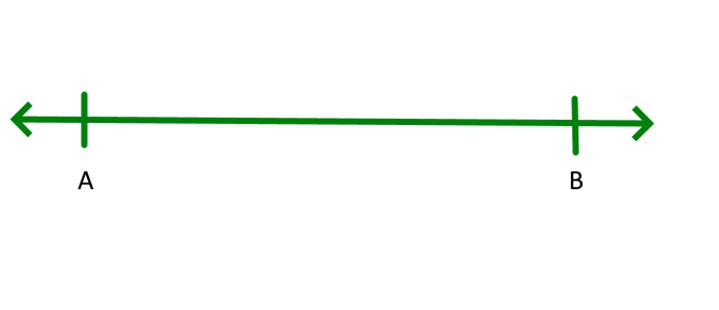### Ray

A ray has a starting point and moves to infinity in one direction.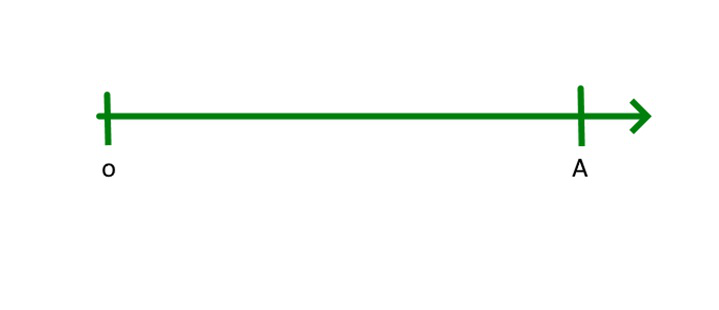Lines based on the concept of intersection

1. Parallel lines
2. Perpendicular lines
3. Transversal line

### Perpendicular Lines

When two lines form a right angle to each other and meet at a single point then they are called perpendicular lines.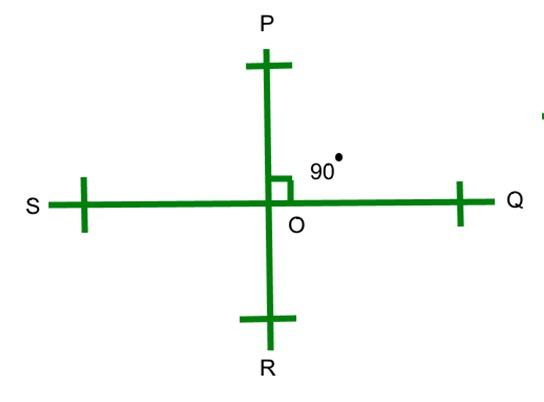### Parallel Lines

Parallel lines are those lines that do not meet each other on a plane at any point and do not intersect with each other.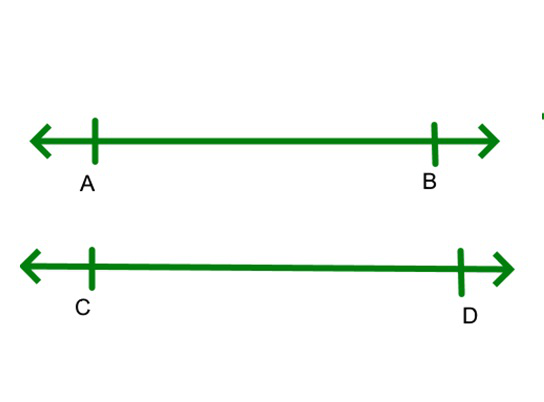### Transversal Lines

When two given lines intersect each other at a distinct point, they will be called transversal lines.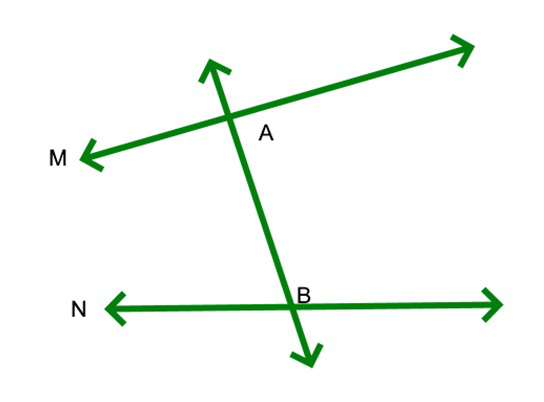Properties of Lines:

• When more than three points lie in the same plane then it will be called collinear points.
• When more than three points, do not lie in the same plane then it will be called non – collinear points.

### Angles

Angles can be categorized as:

Acute Angle: When the angle is less than a right angle, then it will be an acute angle.

Obtuse Angle: When the angle is more than a right angle, then it will be an obtuse angle.

Right Angle: When angle is 90 degrees, then it will be a right angle.

Straight angle: When 180 degrees, an angle is formed, then it will be the straight angle.

Angles based on two concepts, conceptual wise they are

1. Supplementary angles
2. Complementary angles
4. Vertically opposite angles

### Complementary Angles

When two angle sum to 90°, will be a complementary angle.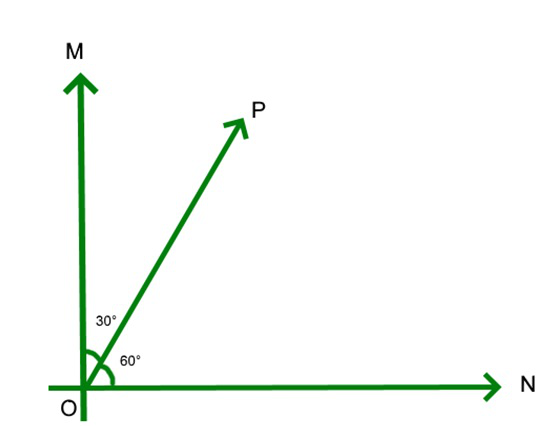### Supplementary Angles

Two angles sum up to 180° will be supplementary angles.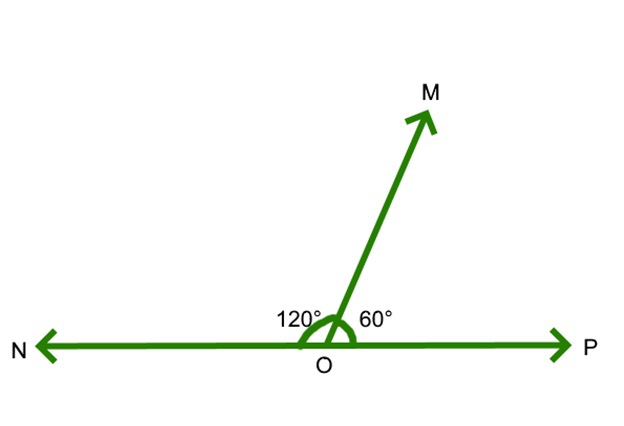When two angles have a common side and a common vertex then the angle called adjacent angles.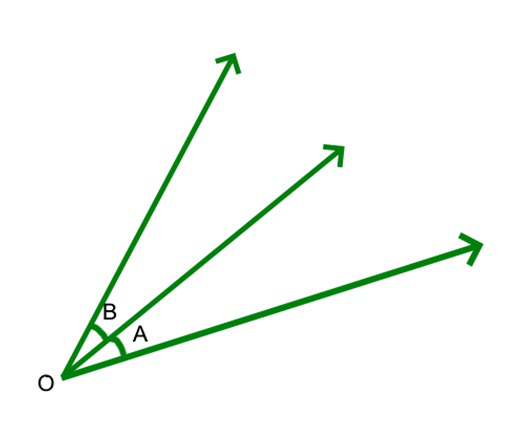### Vertically Opposite Angles

When two angles which are opposite two each other and two lines intersect to each other at a common point are called vertically opposite angle.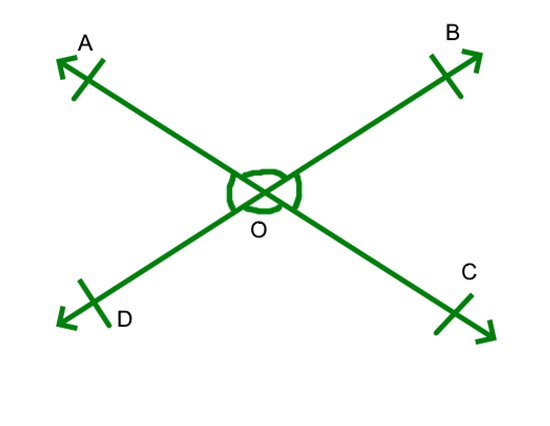∠AOD= ∠BOC

∠AOB= ∠DOC

### Vertical Angles are Congruent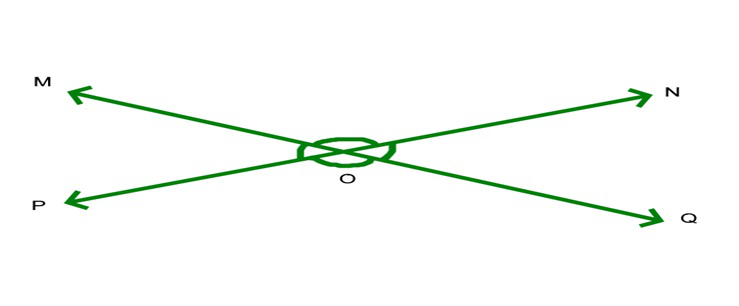Proof:

∠MON= ∠POQ

∠MOP=∠NOQ

Step 1: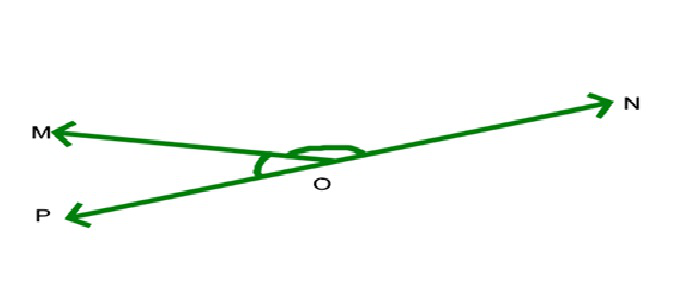∠MOP+ ∠MON≡ 180°

Step 2: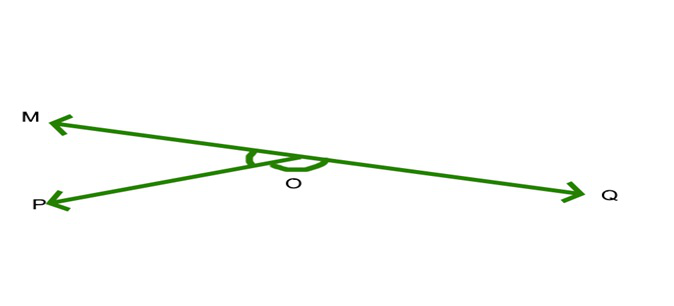∠MOP+ ∠POQ≡ 180°

Therefore: ∠MOP+ ∠MON≡∠MOP+ ∠POQ

∠MOP+ ∠MON-∠MOP≡∠MOP+ ∠POQ-∠MOP

So; ∠MON≡∠POQ

Step 3: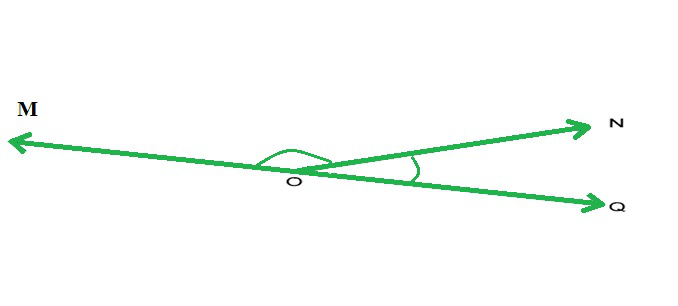∠MON+ ∠NOQ≡ 180°

Step 4: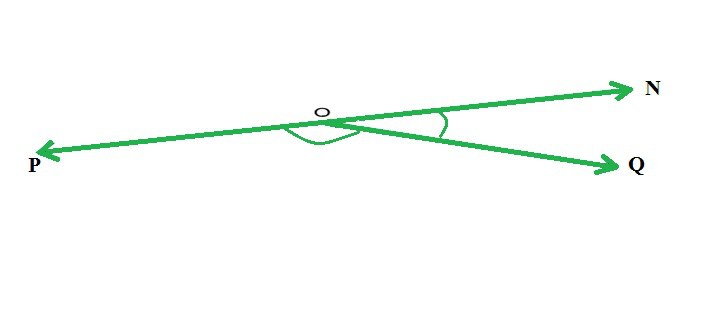∠POQ+ ∠NOQ≡ 180°

Therefore:∠MOP+ ∠NOQ≡∠POQ+ ∠NOQ

So, ∠MOP+ ∠NOQ -∠NOQ≡∠POQ+ ∠NOQ-∠NOQ

∠MOP≡∠POQ

SO: ∠MON= ∠POQ

∠MOP=∠NOQ

### Angles in Triangle sum up to 180°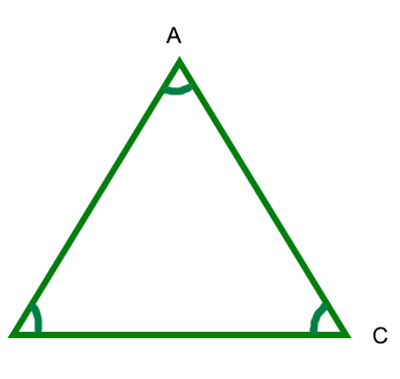A + B + C = 180°

Proof: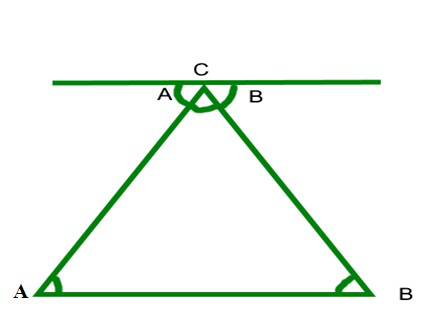As you can see the top line running parallel to the base of triangle

So: ∠ A is the same

∠B is the same

And you can see angle A + B + C does a complete rotation from one side of straight line to the other or 180°

### Parallel lines and Corresponding angles

Corresponding Angles:

Suppose that N, M, and O are distinct lines.  Then N and M are parallel if and only if corresponding angles of the intersection of N and O, and M and O are equal.

Proof: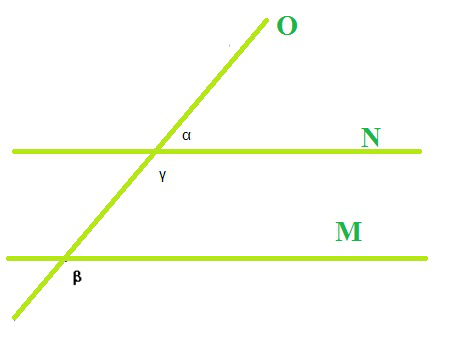=> Assume N and M are parallel, prove corresponding angles are equal.

Assuming N||M, let’s label a pair of corresponding angles α and β.  We know that angle γ is supplementary to angle α from the straight angle theorem (because ZO is a line, and any point on O can be considered a straight angle between two points on either side of the point in question).  Note that β and γ are also supplementary since they form interior angles of parallel lines on the same side of the transversal O (from Same Side Interior Angles Theorem).

Therefore, since γ = 180 – α = 180 – β, we know that α = β.  This can be proven for every pair of corresponding angles in the same way as outlined above.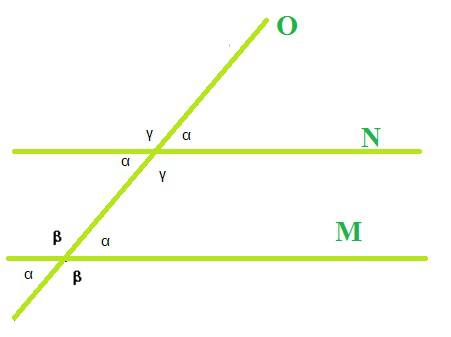<= Assume corresponding angles are equal and prove N and M are parallel.

Assuming corresponding angles, let’s label each angle α and β appropriately.  By the straight angle theorem, we can label every corresponding angle either α or β.

For example, we know α + β = 180º on the right side of the intersection of N and O, since it forms a straight angle on O. Consequently, we can label the angles on the left side of the intersection of N and O α or β since they form straight angles on N.

Since, as we have stated before, α + β = 180º, we know that the interior angles on either side of O add up to 180º.  By the same side interior angles theorem, this makes N || M.

My Personal Notes arrow_drop_up